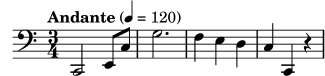### 1.2.1 简单记谱法

LilyPond 会自动添加一些记谱元素。下面的例子中，我们只指定了四个音高，但是 LilyPond 已经为我们加上了谱号，拍子记号和节奏。

```{
c' e' g' e'
}
```#### 音高

Music Glossary: pitch, interval, scale, middle C, octave, accidental.

LilyPond 用小写字母表示音高。 本节中所有例子中的音名都用荷兰命名系统（对应钢琴上的白键 c-b）. 然而 LilyPond 也支持很多不同的命名方法，比如英语，或者唱名 ‘Fixed-Do’ (do-re-mi-...). See Note names in other languages. 字母 `c` 到 `b` 表示 中央 C 下面的一个 ‘小八度’。加上 `'` 或者 `,` 的后缀表示高八度或低八度。 下面是一段从 middle C 开始的音阶和琶音：

```{ c' d' e' f' g' a' b' c'' g c' e' g' c'' e'' g'' c''' }
``````\relative {
c' d e f
g a b c
}
```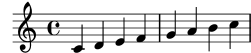```\relative {
d' f a g
c b f d
}
```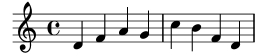```\relative {
e'' c a c
}
```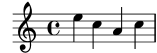```\relative {
b' c % c is 1 staff space up, so is the c above
b d  % d is 2 up or 5 down, so is the d above
b e  % e is 3 up or 4 down, so is the e above
b a  % a is 6 up or 1 down, so is the a below
b g  % g is 5 up or 2 down, so is the g below
b f  % f is 4 up or 3 down, so is the f below
}
```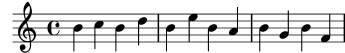```\relative {
a' a, c' f,
g g'' a,, f'
}
```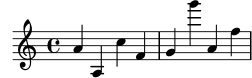#### 时值 (节奏)

```\relative {
a'1
a2 a4 a8 a
a16 a a a a32 a a a a64 a a a a a a a a2
}
``````\relative {
a'4 a a4. a8
a8. a16 a a8. a8 a4.
}
```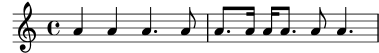#### 休止符

```\relative {
a'4 r r2
r8 a r4 r4. r8
}
```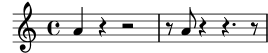#### 拍子记号

```\relative {
\time 3/4
a'4 a a
\time 6/8
a4. a
\time 4/4
a4 a a a
}
```#### 速度记号

```\relative {
\time 3/4
\tempo "Andante"
a'4 a a
\time 6/8
\tempo 4. = 96
a4. a
\time 4/4
\tempo  "Presto" 4 = 120
a4 a a a
}
```#### 谱号（音部记号）

```\relative {
\clef "treble"
c'1
\clef "alto"
c1
\clef "tenor"
c1
\clef "bass"
c1
}
```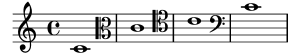#### 所有的都放在一起

```\relative {
\clef "bass"
\time 3/4
\tempo "Andante" 4 = 120
c,2 e8 c'
g'2.
f4 e d
c4 c, r
}
```